## 1 基础知识

XGBoost的成功可以总结为回归（树回归+线性回归）+提升（boosting）+优化（5个方面）牛顿法、预排序、加权分位数、稀疏矩阵识别以及缓存识别等技术来大大提高了算法的性能。下面开始介绍一些入门必须的基础知识：

### 1.2 低维到高维的转变

#### 梯度和Hessian矩阵

• 二阶导数和Hessian矩阵

• 输入为标量的泰勒级数展开
• 输入为向量的泰勒级数展开

### 1.4 极值点

• 输入为标量的泰勒展开
• 严格局部极小点指：$f(x_k + \delta) > f(x_k)$

• 称满足$f’(x_k) = 0$的点为平稳点（候选点）。

• 函数在$x_k$有严格局部极小值条件为$f’(x_k) = 0$且$f’’(x_k) > 0$。

• 输入为向量的泰勒级数展开
• 称满足$g(x_k) = 0$的点为平稳点（候选点），此时如果有

$H(x_k) \succ 0$， $x_k$为一个严格局部极小点（反之，局部严格最大点）
如果$H(x)$不定矩阵，是一个鞍点(saddle point)。（如下图所示）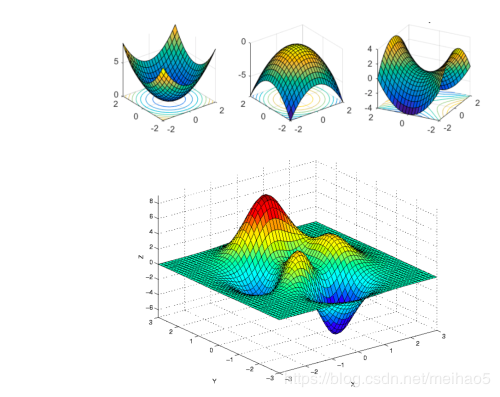### 1.5 怎么求一个函数的极值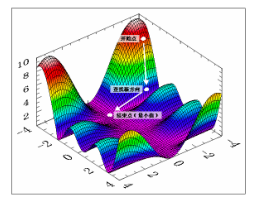Xgboost也是GBDT的一中，只不过进行了大量的优化！，其中一点就是优化方法选取了牛顿法（选取的方向不一样，一个梯度的方向，一个二阶导数的方向）

## 2 GBDT(梯度提升树）

**梯度提升算法的本质：拟合一个回归树是的损失函数最小。

### 2.1 参数空间与函数空间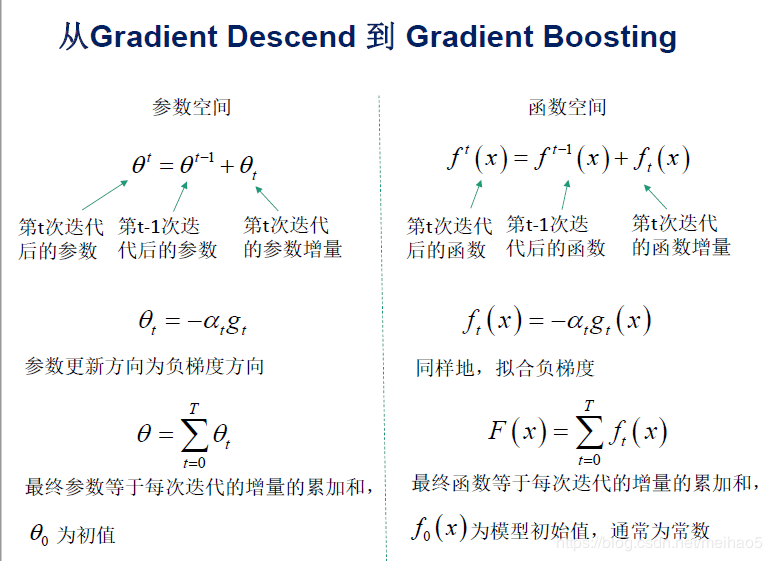### 2.2 Boosting思想

Gradient Boosting Tree 算法原理

• Friedman在论文greedy function approximation: a gradient boosting machine中提出GBDT。

• 其模型F定义为加法模型：

其中，x 为输入样本， h 为分类回归树，w 是分类回归树的参数，$\alpha$ 是每个树的权重。

• 通过最小化损失函数求解最优模型：

NP难问题 -> 通过贪心算法，迭代求局部最优解。

1. 初始化$f_0$
2. for t=1 to T do
2.1 计算响应：
$\tilde y_i = -[\frac{\partial L(y_i, F(x_i))}{\partial F(x_i)}]_{F(x) = F_{t-1}(x)}, i = 1,2,\cdots,N$
2.2 学习第t棵树：
$w^ = \mathop{\arg\min}\limits_{w} \sum_{i=1}^N(\tilde y_i - h_t(x_i;w))^2$
2.3 line search 找步长（前向分步算法）：
$\rho^ = \mathop{\arg\min}\limits_{\rho} \sum_{i=1}^N L(y_i,F_{t-1}(x_i) + \rho h_t(x_i;w^))$
2.4 令$f_t = \rho^ h_t(x;w^*)$，更新模型：
$F_t = F_{t-1} + f_t$
3. 输出$F_T$

2.1 计算残差（计算值域真实值之间的误差）
2.2 拟合是的残差最小（当前学习的这棵树）
2.3 $\rho$步长：基于学习器的权重， H树：表示方向
2.4 得到当前这一步的树

### 2.3 如果要将GBDT用于分类问题，怎么做呢？

$p1(x_i)=\frac{exp(F1(x_i))}{\sum_{i=1}^3 (F1(xi))}$

## 3 XGBoost

### 3.1 模型函数形式

$q(x)$ 表示将样本 x 分到了某个叶子结点上， w 是叶子结点的分数(leaf score)， 所以 $w_{q(x)}$ 表示回归树对样本的预测值。

### 3.2 目标函数

• $L(\Theta)$是误差函数，衡量模型拟合数据的程度；
• $\Omega (\Theta)$ 是正则化项，用来惩罚复杂模型的。

• Ridge Regression （岭回归）： $\sum_{i=1}^n (y_i - \theta^T x_i)^2 + \lambda ||\theta||^2$
• LASSO：$\sum_{i=1}^n (y_i - \theta^T x_i)^2 + \lambda ||\theta||_1$

### 3.3 正则项

• 通过偏差方差分解去解释
• PAC-learning 泛化界解释
• Bayes 先验解释，把正则当成先验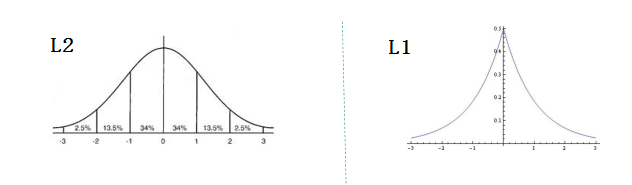• L2 正则中，模型参数服从搞死分布 $\theta ~ N(0,\sigma^2)$， 对参数加了分布约束，大部分绝对值很小；
• L1 正则中， 模型参数服从拉普拉斯分布， 对参数加了分布约束，大部分取值为0。

### 3.4 XGBoost 的目标函数

#### 正则项

XGBoost的目标函数（函数空间）：

XGBoost采用的是：

GBDT 是通过求一阶导数，迭代法的方式在函数空间拟合一个最小值。 XGBoost 通过泰勒展开实现了更精确的拟合。

### 3.5 误差函数的二阶泰勒展开

• 第 t 次迭代后， 模型的预测等于前 t-1 次的模型预测加上第 t 颗树的预测：

• 此时目标函数可写作：

公式中 $y_i, \tilde y_i^{(t-1)}$都已知， 模型要学习的只有第 t 颗树$f_t$

• 将误差函数在 $\tilde y_i^{(t-1)}$ 处二阶泰勒展开：

• 将公式中的常数项去掉，得到：

• 把 $f_t, \Omega(f_t)$ 写成树结构的形式， 即把下式带入目标函数中：

• $\sum_{i=1}^n [g_i w_{q(x_i)} + \frac{1}{2} h_i w_{q(x_i)}^2]%=$ 是对样本的累加， $\frac{1}{2} \sum_{j=1}^T w_j^2$ 是对叶节点的累加。

• 如何统一呢？定义每个叶节点 j 上的样本集合为 $I_j = \{ i | q(x_i) = j \}$，则目标函数可以写成按叶节点累加的形式：

• 如果确定了树的结构（即 $q(x)$ 确定了）， 为了使目标函数最小，可以令其导数为0， 解得每个叶节点的最优预测分数为：

### 3.6 回归树的学习策略

• 暴力枚举所有可能的树结构，选择损失值最小的 - NP 难问题
• 贪心法， 每次尝试分裂一个叶节点，计算分裂前后的增益，选择增益最大的。

• ID3 算法采用信息增益
• C4.5 算法采用信息增益比
• CART 采用 Gini 系数
• XGB 不一致

### 3.7 XGBoost 的打分函数

Gain 值越大，分裂后 L 减小越多。所以当对一个叶节点分割时，计算所有候选（feature, value）对应的 gain， 选取 gain 最大的进行分割。

### 3.8 树节点分裂算法

• 近似算法距离：三分位数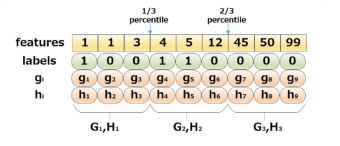• 实际上 XGBoost 不是简单按照样本个数进行分位， 而是以二阶导数值作为权重（Weighted Quantile Sketch）， 比如：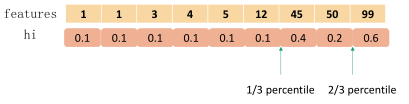• 为什么用 $h_i$ 加权，就是把目标函数整理成以下形式，可以看出 $h_i$ 有对 loss 加权的作用。

### 3.9 稀疏值处理

• 稀疏值：缺失导致，诸如类别类 one-hot 编码会导致大量 0 值出现。
• 当特征出现缺失值的时候 XGBoost 可以学习出默认的节点分裂方向，如下图算法所示：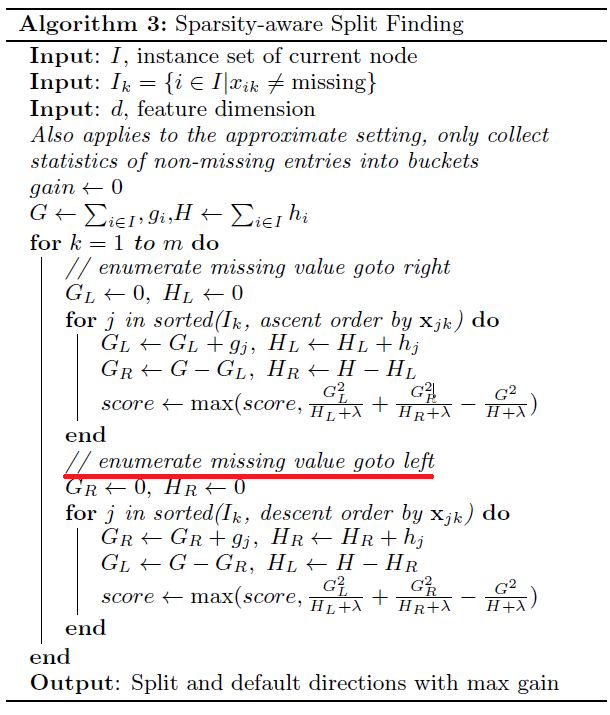#### 最后一步：

Shrinkage论文提到：关于 n 和迭代次数 T 的取值，可以通过交叉验证得到合适的值，通常针对不同问题，其具体值是不同的。一般来说，当条条件允许时（如对模型训练时间没有要求等）可以设置一个较大的迭代次数 T ，然后针对该 T 值利用交叉验证来确定一个合适的 n 值。但 n 的取值也不能太小，否则模型达不到较好的效果.

## 4 更多特性

### 4.1 XGBoost 的其他特性

• 行抽样（row sample）
• 列抽样（column sample），借鉴随机森林
• Shrinkage（缩减）， 即学习速率
将学习速率调小，迭代次数增多，有正则化作用
• 支持自定义损失函数（需二阶可导）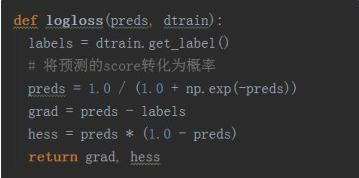### 4.2 XGBoost 的系统设计

• Column Block
1. 特征预排序，以 column block 的结构存于内存中
2. 存储样本索引（instance indices）
3. block 中的数据以稀疏格式（CSC）存储

• Cache Aware Access
1. column block 按特征大小顺序存储， 相应的样本的梯度信息是分散的，造成内存的不连续访问，降低 CPU cache 命中率
2. 缓存优化方法
• 预取数据到buffer 中（非连续->连续）， 在统计梯度信息
• 调节块的大小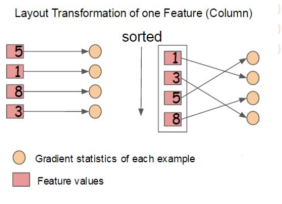### 4.3 更高效的工具包 LightGBM

• 速度更快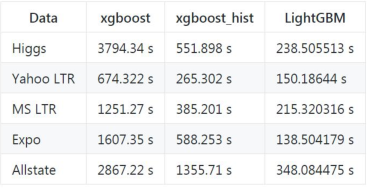• 内存占用更低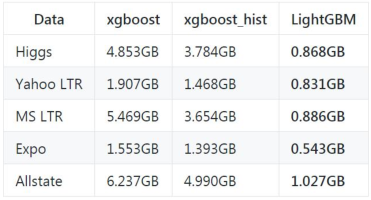• 准确率更高（优势不明显， 与 XGBoost 相当）
在微软的论文中说是改动很大，实际应用中没有那么明显，可能与数据集有关系

### 4.4 XGBoost 的参数意义与调优：

1）Booster: 分类器类型
2）lambda: 正则化
3）min_child_weight:子节点权重
4）树的深度
4）学习率n
……

## XGBoost总结：

1. 损失函数是用泰勒展式二项逼近，而不是像GBDT里的就是一阶导数；
2. 对树的结构进行了正则化约束，防止模型过度复杂，降低了过拟合的可能性；
3. 实现了并行化（树节点分裂的时候）
4. 开头提到的优化
5. 回归模型可选

-------------本文结束感谢您的阅读-------------